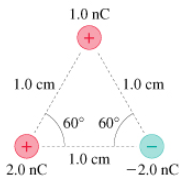# Problem: What is the force F? on the 1.0 nC charge in (Figure 1)?A) Determine the magnitude of the force.B) Determine the direction of the force.

###### FREE Expert Solution

k = 9 × 109 N•m2.C2

d =

99% (166 ratings)###### Problem DetailsWhat is the force F? on the 1.0 nC charge in (Figure 1)?

A) Determine the magnitude of the force.

B) Determine the direction of the force.